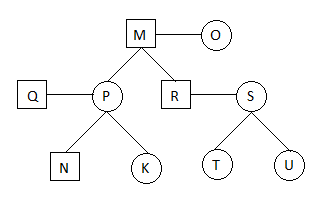# Blood Relation Quiz With Solution For SBI PO Mains: Part 1Directions (Q.1-5): Read the information given below and answer the questions that follow.
I. M, P, S, T, R, Q, O, K, U and N are the 10 members of a family.
II. P is the daughter of M and the sister of R.
III. Q is the son in law of O.
IV. M is the grandfather of T and U.
V. P is the sister in law of S.
VI. R is the maternal uncle of N and K.
VII. N is the only son of Q.
VIII. T and U are the sisters.

Q.1 How is P related to T?
A. Daughter
B. Sister
C. Aunt
D. Mother
E. None of these.

Q.2 Which of the following is true?
A. R is the sister of P.
B. K is the son of S.
C. O is the husband of M.
D. S is the daughter in law of M.
E. None of these.

Q.3 How many couples are there in the family?
A. 3
B. 4
C. 5
D. 1
E. 2

Q.4 What is R's relation with Q?
A. Son in law
B. Husband
C. Wife
D. Sister in law
E. Brother in law

Q.5 How many male members in the family?
A. 5
B. 6
C. 3
D. 2
E. 4

1. C. Aunt
2. D. S is the daughter in law of M.
3. A 3
4. E Brother-in-law
5. E 4

## Solution